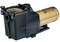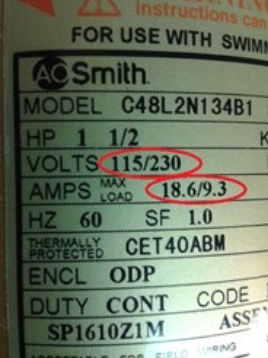This guide illustrates how easy it is to calculate the cost of running a pool pump. One of the most powerful consumers of energy in homes with swimming pools are pool pumps. With the rising electric costs, it is important to understand how much it costs to run a pool pump.

1. Locate the voltage and amperage rating of the pump. The specifications should be listed on the motor label. In our example we used a 1.5HP Hayward Super Pump. The voltage rating is 115/230 and the amp rating is 18.6/9.3. If you run a pump on the lower voltage rating (115) you will select the higher amperage rating (18.6). If you run the pump on the higher voltage (230) you will select the lower amperage rating (9.3). For this example we will use the 230 voltage and 9.3 amperage rating.2. Multiply the voltage rating by the amperage rating. This will give the total watts. Example: 230 volt X 9.3 amps = 2,139 watts

3. Divide the total watts by 1,000 to get kilowatts (kW). Example: 2,139 watts / 1,000 = 2.139 kW

4. Multiply the hours per day you run the pool pump by the kW. This total will be the kWh per day. Example: 8 hours/day X 2.139 kW = 17.112 kWh per day.

5. Multiply the kWh per day by the cost per kWh. The cost per kWh can be found on your most recent power bill. The result will tell you how much it costs to run your pump each day. Example: 17.112 kWh a day x .12 cents per kWh = \$2.05 per day to operate the pool pump.

If you have any other questions about pool and spa products please do let us know - we are here to help!